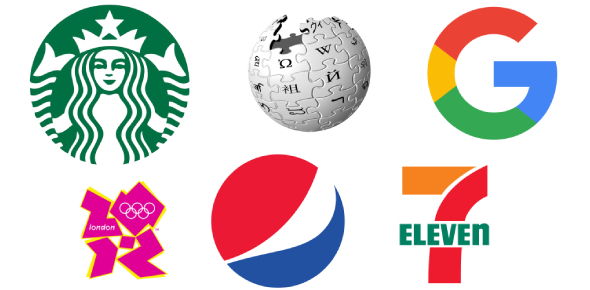# Perry Science 1 Chapter 1 Retest

44 Questions | Total Attempts: 91SettingsRetest Science Spectrum Balanced Approach Chapter 1

• 1.
You are asked to find the area of a room that is 4.56 m long and 5.668 m wide.  How many significant figures should you show in your answer?
• A.

5

• B.

3

• C.

7

• D.

6

• 2.
Scientific theoris can be changed or replaced when
• A.

Scientists make models of events or objects

• B.

• C.

New technology is invented

• D.

Scientists decide to work on different problems

• 3.
Using the line graph on the screen, at what time of day  was the temperature approximately 4C?
• A.

12:00 PM

• B.

11:00 AM

• C.

10:00 AM

• D.

9:00 AM

• 4.
Using the line graph on the screen, at which two times of day was teh temperature the same?
• A.

7:00 AM and 7:00 PM

• B.

7:00 AM and 10:00 PM

• C.

10:00 AM and 10:00 PM

• D.

10:00 AM and 7:00 PM

• 5.
The two main branches of science are
• A.

Natural and physical science

• B.

Physics and chemistry

• C.

Natural and social science

• D.

Biology and earth science

• 6.
What does it mean to say that "no experiment is a failure?"
• A.

All experiments yield the desired results

• B.

All experiments are observations of real events

• C.

All experiments give scientists work to do

• D.

All experiments involve manipulating variables

• 7.
A precise measurement is one that
• A.

Contains the correct number of significant figures.

• B.

Is as exact as possible.

• C.

Is close to the true value.

• D.

Contains at least three significant digits.

• 8.
Using the bar graph on the screen,  for which year was the smallest number of pennies found?
• A.

1990

• B.

1991

• C.

1989

• D.

1988

• 9.
The sample contained the same number of pennies for which two years?
• A.

1988 and 1991

• B.

1988 and 1992

• C.

1994 and 1998

• D.

1994 and 1997

• 10.
The speed of light is approximately 3 x 108 m/s.  how would this be written in conventional notation?
• A.

300,000 m/s

• B.

300,000,000 m/s

• C.

30,000,000 m/s

• D.

3,000,000 m/s

• 11.
The first step in the scientivic method is usually
• A.

Making an observation

• B.

Forming a hypothesis

• C.

Testing a hypothesis

• D.

Collecting data

• 12.
A loaf of bread weighs 1362 g. The weight in kilograms is
• A.

001362 kg

• B.

1362 kg

• C.

1.362 kg

• D.

01362 kg

• 13.
A scientific theory is an explanation that
• A.

Has been published in a journal or book.

• B.

Predicts what will happen

• C.

Has been tested by many observations

• D.

A scientist has tested with an experiment

• 14.
A series of logical steps that is followed in order to solve a problem is called the
• A.

Experimental process

• B.

Scientific theory

• C.

Model method

• D.

Scientific method

• 15.
What  do scientists who do pure science do?
• A.

They look for ways to use scientific knowledge to solve problems.

• B.

They do experiments to find out about the world.

• C.

They build faster and more powerful computers.

• D.

They develop new uses for scientific knowledge.

• 16.
The decimal equivalent of  10-2 is
• A.

0.1

• B.

10

• C.

0.01

• D.

100

• 17.
Scientists use computer models to study complicated events and to
• A.

Perform experiments

• B.

Make predictions

• C.

Change theories and laws

• D.

State theories

• 18.
What is 78,900,000,000 expressed in scientific notation?
• A.

7.89 x10^11

• B.

7.89 x10^10

• C.

7.89 x10^9

• D.

789 x 10^9

• 19.
Which question cannot be answered by an experiment?
• A.

Did a comet impact kill the dinosaur?

• B.

• C.

Is rabies caused by a virus?

• D.

Does penicillin kill Salmonella bacteria?

• 20.
Scientists test a hypothesis by
• A.

Doing experiments

• B.

Designing models

• C.

Formulating questions

• D.

Drawing conclusions

• 21.
Technology can be best defined as
• A.

The use of lenses and microscopes

• B.

New inventions

• C.

The use of scientific discoveries

• D.

Science that uses computers

• 22.
The force with which gravity pulls on a quantity of matter is referred to as
• A.

Weight

• B.

Length

• C.

Mass

• D.

Volume

• 23.
The main branches of natural science are
• A.

Biology, zoology, and ecology

• B.

Medicine and agriculture

• C.

Life, physical, and earth science

• D.

Physics and chemistry

• 24.
Maria is 123 centimeters tall.  Her height in meters is
• A.

0.123 m.

• B.

0123 m

• C.

1.23 m

• D.

12.3 m

• 25.
A measurement that is accurate is one that
• A.

Contains five decimal places

• B.

Contains at least four significant digits

• C.

Is as exact as possible

• D.

Is close to the true value

Related TopicsBack to top